## Example Questions

← Previous 1

### Example Question #3 : How To Find The Volume Of A Sphere

For a sphere the volume is given by = (4/3)πr3 and the surface area is given by = 4πr2. If the sphere has a surface area of 256π, what is the volume?

300π

750π

615π

683π

683π

Explanation:

Given the surface area, we can solve for the radius and then solve for the volume.

4πr2 = 256π

4r2 = 256

r2 = 64

r = 8

Now solve the volume equation, substituting for r:

V = (4/3)π(8)3

V = (4/3)π*512

V = (2048/3)π

V = 683π

### Example Question #9 : How To Find The Volume Of A Sphere

The specifications of an official NBA basketball are that it must be 29.5 inches in circumference and weigh 22 ounces.  What is the approximate volume of the basketball?   Remember that the volume of a sphere is calculated by V=(4πr3)/3

3468.05 cu.in.

434.19 cu.in.

92.48 cu.in.

138.43 cu.in.

8557.46 cu.in.

434.19 cu.in.

Explanation:

To find your answer, we would use the formula:  C=2πr. We are given that C = 29.5. Thus we can plug in to get  [29.5]=2πr and then multiply 2π to get 29.5=(6.28)r.  Lastly, we divide both sides by 6.28 to get 4.70=r. Then we would plug into the formula for volume V=(4π〖(4.7)〗3) / 3   (The information given of 22 ounces is useless)

### Example Question #1 : How To Find The Volume Of A Sphere

The radius of a sphere is. What is the approximate volume of this sphere?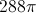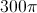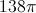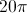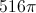Explanation: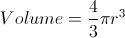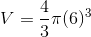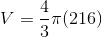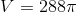### Example Question #1 : How To Find The Volume Of A Sphere

A cube has a side dimension of 4. A sphere has a radius of 3. What is the volume of the two combined, if the cube is balanced on top of the sphere?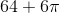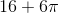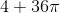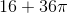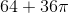Explanation: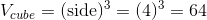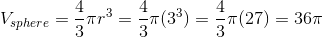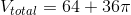### Example Question #3 : Spheres

What is the volume of a sphere with a diameter of 6 in?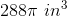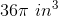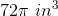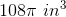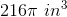Explanation:

The formula for the volume of a sphere is: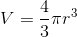where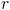= radius.  The diameter is 6 in, so the radius will be 3 in.

### Example Question #11 : How To Find The Volume Of A Sphere

If the diameter of a sphere is, find the approximate volume of the sphere?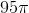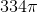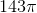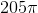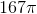Explanation:

The volume of a sphere =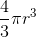Radius isof the diameter so the radius = 5.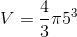or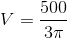which is approximately### Example Question #4 : How To Find The Volume Of A Sphere

What is the volume of a sphere with a diameter ofinches? Leave your answer in terms of.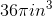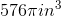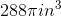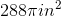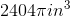Explanation:

To find the volume of a sphere we use the sphere volume formula:First we need to find the radius of the sphere. A sphere has a radius of half the diameter. So we see that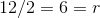.

Next we plug 6 in for our radius and get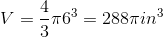### Example Question #5 : How To Find The Volume Of A Sphere

What is the volume of a sphere with a diameter of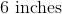(reduce all fractions)?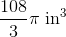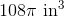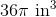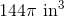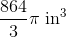Explanation:

The formula for the volume of a sphere is: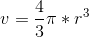, thus we need to just determine the radius and plug it into the equation. Remember that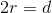and so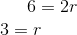And plugging in we get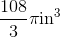### Example Question #6 : How To Find The Volume Of A Sphere

What is the volume of a sphere with a surface area of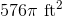? (Simplify all fractions in your answer.)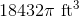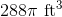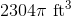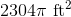Explanation:

First find the radius from the surface area set the given surface area equal to the surface area formula and solve for the radius.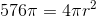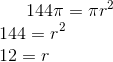Now plug the radius into the volume formula: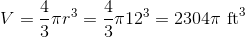### Example Question #7 : How To Find The Volume Of A Sphere

If Ariana’s orange has twice the radius of Autumn’s orange, the volume of Ariana’s orange is how many times larger than the volume of Autumn’s orange?Define the radius of Autumn’s orange as r. The volume of her orange is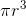. Ariana’s orange has twice the radius of Autumn’s, so the radius of her orange is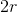, and the volume is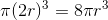, which is 8 times larger than Autumn’s orange.